## 12.4 The fractals module

fractals.julia(,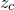,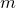)
The fractals.julia(,,) function tests whether the pointin the complex plane lies within the Julia set associated with the pointin the complex plane. The expression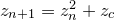is iterated until either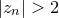, in which case the iteration is deemed to have diverged, or untiliterations have been exceeded, in which case it is deemed to have remained bounded. The number of iterations required for divergence is returned, oris returned if the iteration remained bounded – i.e. the point lies within the numerical approximation to the Julia set.

fractals.mandelbrot(,)
The fractals.mandelbrot(,) function tests whether the pointin the complex plane lies within the Mandelbrot set. The expression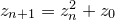is iterated until either, in which case the iteration is deemed to have diverged, or untiliterations have been exceeded, in which case it is deemed to have remained bounded. The number of iterations required for divergence is returned, oris returned if the iteration remained bounded – i.e. the point lies within the numerical approximation to the Mandelbrot set.